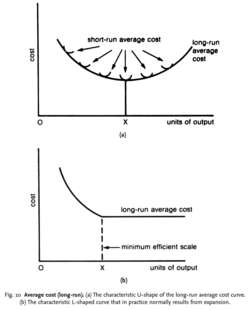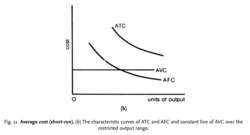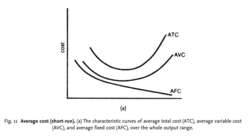# average cost

(redirected from Average total cost)
Also found in: Dictionary, Thesaurus, Medical, Acronyms.
Related to Average total cost: marginal cost, Total fixed cost

## Average cost

In the context of investing, refers to the average cost of shares or stock bought at different prices over time.

## Average Cost

1. A method of determining the value of securities in a tax year. One calculates the average cost by taking the total cost of buying shares in a security and dividing it by the number of shares one owns. The average-cost method is useful especially when the security has fluctuated significantly in price and when the investor has an automatic investment plan.

2. In inventory, a method to determine the value of one unit. It is calculated by dividing the total cost of buying the inventory by the units available for sale. See also: Inventory Valuation.

## average cost

A cost amount calculated by dividing the total cost by the units of production. Thus, if a firm produces 10,000 units of output for a total cost of \$25,000, the average cost of each unit is \$25,000/10,000 units, or \$2.50 per unit. Average cost is made up of costs remaining unchanged throughout a range of output and costs varying directly with output. Firms with the lowest average cost in an industry have a competitive advantage in the event of severe competition and price cutting.

## average cost

the unit cost of a product (total cost divided by number of units produced). Average total cost (ATC) can be split up into average FIXED COST (AFC) and average VARIABLE COST (AVC). AFC declines continuously as output rises, as a given total amount of fixed cost is ‘spread’ over a greater number of units. For example, with fixed costs of £1,000 per year and annual output of 1000 units, fixed costs per unit would be £1, but if annual output rose to 2000 units the fixed cost per unit would fall to 50p. Unit variable cost (AVC) tends to remain constant over the output range.
Collins Dictionary of Business, 3rd ed. © 2002, 2005 C Pass, B Lowes, A Pendleton, L Chadwick, D O’Reilly and M AffersonFig. 10 Average cost (long-run). (a) The characteristic U-shape of the long-run average cost curve.

(b) The characteristic L-shaped curve that in practice normally results from expansion.

## average cost (long-run)

the unit cost (TOTAL COST divided by number of units produced) of producing outputs for plants of different sizes. The position of the SHORT-RUN average total cost (ATC) curve depends on its existing size of plant. In the long run, a firm can alter the size of its plant. Each plant size corresponds to a different U-shaped short-run ATC curve. As the firm expands its scale of operation, it moves from one curve to another. The path along which the firm expands - the LONG-RUN ATC curve - is thus the envelope curve of all the possible short-run ATC curves.

It will be noted that the long-run ATC curve is typically assumed to be a shallow U-shape, with a least-cost point indicated by output level OX. To begin with, average cost falls (reflecting ECONOMIES OF SCALE); eventually, however, the firm may experience DISECONOMIES OF SCALE and average cost begins to rise.

Empirical studies of companies’ long-run average-cost curves, however, suggest that diseconomies of scale are rarely encountered within the typical output ranges over which companies operate, so that most companies’ average cost curves are L-shaped, as in Fig. 10 (b). In cases where diseconomies of scale are encountered, the MINIMUM EFFICIENT SCALE at which a company will operate corresponds to the minimum point of the long-run average cost curve (Fig. 10 (a)). Where diseconomies of scale are not encountered within the typical output range, minimum efficient scale corresponds with the output at which economies of scale are exhausted and constant returns to scale begin (Fig. 10 (b)). Compare AVERAGE COST (SHORT-RUN).Fig. 11 Average cost (short-run). (b) The characteristic curves of ATC and AFC and constant line of AVC over the restricted output range.Fig. 11 Average cost (short-run). (a) The characteristic curves of average total cost (ATC), average variable cost (AVC), and average fixed cost (AFC), over the whole output range.

## average cost (short-run)

the unit cost (TOTAL COST divided by the number of units produced) of producing particular volumes of output in a plant of a given (fixed) size. Average total cost (ATC) can be split up into average FIXED COST (AFC) and average VARIABLE COST (AVC). AFC declines continuously as output rises as a given total amount of fixed cost is ‘spread’ over a greater number of units. For example, with fixed costs of £1,000 per year and annual output of 1,000 units, fixed costs per unit would be £1, but if annual output rose to 2,000 units, the fixed cost per unit would fall to 50 pence - see AFC curve in Fig. 11 (a).

Over the whole potential output range within which a firm can produce, AVC falls at first (reflecting increasing RETURNS TO THE VARIABLE FACTOR INPUT output increases faster than costs), but then rises (reflecting DIMINISHING RETURNS to the variable inputs - costs increase faster than output), as shown by the AVC curve in Fig. 11 (a). Thus the conventional SHORT-RUN ATC curve is U-shaped.

Over the more restricted output range in which firms typically operate, however, constant returns to the variable input are more likely to be experienced, where, as more variable inputs are added to the fixed inputs employed in production, equal increments in output result. In such circumstances, AVC will remain constant over the whole output range, as in Fig. 11 (b), and as a consequence ATC will decline in parallel with AFC. Compare AVERAGE COST (LONG-RUN). See LOSS, LOSS MINIMIZATION.

Collins Dictionary of Economics, 4th ed. © C. Pass, B. Lowes, L. Davies 2005
References in periodicals archive ?
At the optimal solutions, (1) R is higher for lost sales (as expected, because in our example, backlog cost and lost sales cost are the same, so it is only natural that the cycle service level would be set higher (less PS) to try to prevent shortages for lost sales), (2) Q is higher for backlog because it must satisfy demands during stockout, (3) higher R leads to higher EO and PO for lost sales, whereas higher Q leads to higher holding cost for backlog, and (4) although backlog has a higher total cost, it also has a longer cycle length, which leads to a lower average total cost per period.
This study provides pertinent information that will allow athletic departments to be sized in a manner that would produce the lowest average total cost per athlete.
6 shows the variation of the average total costs per unit of time and part according to time in the case of [C.sub.r] = 0.75 for various values of [beta].
Repeating the analysis with a hypothetical 50% reduction in the cost of the Gynae Test Kit decreases the average total cost of an LBC smear to R75 (95% CI 69-81), but even this large reduction in cost alone would not be sufficient to outperform conventional cytology, as the average cost per case detected (R9 826; 95% CI 8 082-12 171) would still be higher compared with conventional cytology.
optimization objective: minimize the average total cost (ATC).
Again, when 3600 simulations were performed, we obtained the average solution as follows: [N.sub.70] = 58, the average RUD is 7.7803, and the average total cost is 199.4059.
The average total cost of processing was N20, 250.9, which was dominated by the variable cost of processing which accounted for 90.7% of the average total cost.
Excluding shopping, the average total cost for each trip for a Chinese tourist stands at 2,000 euros.
Country Average Cost Average Total Cost of Per Record (USD) a Breach (USD) Australia \$114 \$1.83 million France \$119 \$2.53 million Germany \$177 \$3.44 million UK \$98 \$2.57 million us \$204 \$6.75 million AVERAGE \$142 \$3.43 million Source: The "2009 Annual Study: Global Cost of a Data Breach" report, compiled by the Ponemon Institute and sponsored by PGP Corporation
The average total cost of a corporate data breach stands at \$6.3 million and lost business accounts for 65% of breach costs, according to a study by the Ponemon Institute.
Although pricing below average total cost and above average variable cost is not inherently predatory, it does not follow however, that such prices are never predatory.
The source added: "The average total cost of care is pounds 30,000.

Site: Follow: Share:
Open / Close